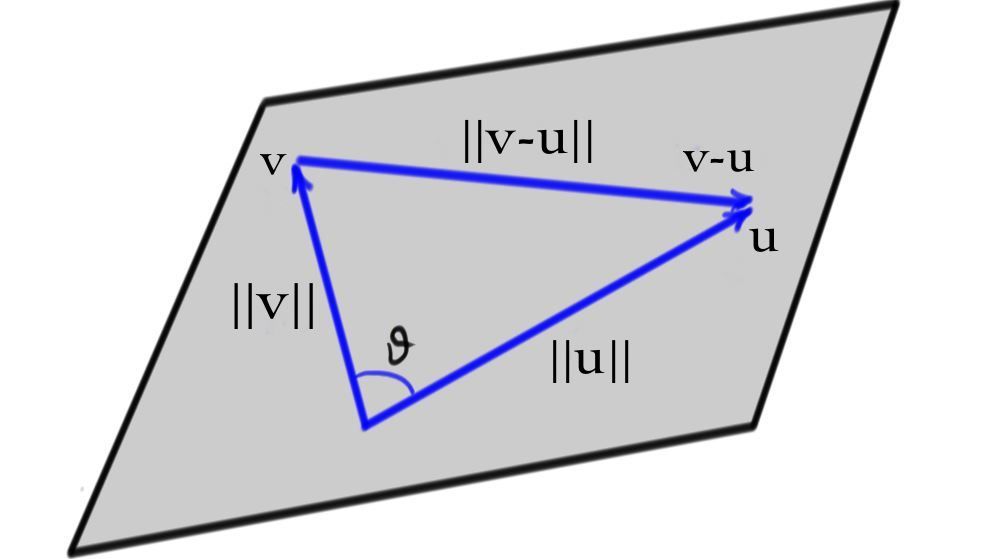$$\newcommand{\id}{\mathrm{id}}$$ $$\newcommand{\Span}{\mathrm{span}}$$ $$\newcommand{\kernel}{\mathrm{null}\,}$$ $$\newcommand{\range}{\mathrm{range}\,}$$ $$\newcommand{\RealPart}{\mathrm{Re}}$$ $$\newcommand{\ImaginaryPart}{\mathrm{Im}}$$ $$\newcommand{\Argument}{\mathrm{Arg}}$$ $$\newcommand{\norm}{\| #1 \|}$$ $$\newcommand{\inner}{\langle #1, #2 \rangle}$$ $$\newcommand{\Span}{\mathrm{span}}$$

# 4.3: Directions and Magnitudes

$$\newcommand{\vecs}{\overset { \rightharpoonup} {\mathbf{#1}} }$$

$$\newcommand{\vecd}{\overset{-\!-\!\rightharpoonup}{\vphantom{a}\smash {#1}}}$$

Consider the $$\textit{Euclidean length}$$ of a vector:

$\|v\| := \sqrt{(v^{1})^{2} + (v^{2})^{2}+\cdots(v^{n})^{2}}\ =\ \sqrt{ \sum_{i=1}^{n} (v^{i})^{2} }\: .$

Using the Law of Cosines, we can then figure out the angle between two vectors. Given two vectors $$v$$ and $$u$$ that $$\textit{span}$$ a plane in $$\mathbb{R}^{n}$$, we can then connect the ends of $$v$$ and $$u$$ with the vector $$v-u$$.Then the Law of Cosines states that:

$\|v-u\|^2 = \|u\|^2 + \|v\|^2 - 2\|u\|\, \|v\| \cos \theta$

Then isolate $$\cos \theta$$:

\begin{eqnarray*}
\|v-u\|^{2} - \|u\|^{2} - \|v\|^{2} &=& (v^{1}-u^{1})^{2} + \cdots + (v^{n}-u^{n})^{2} \\
& & \quad - \big((u^{1})^{2} + \cdots + (u^{n})^{2}\big) \\
& & \quad - \big((v^{1})^{2} + \cdots + (v^{n})^{2}\big) \\
& = & -2 u^{1}v^{1} - \cdots - 2u^{n}v^{n}
\end{eqnarray*}
Thus,

$\|u\|\, \|v\| \cos \theta = u^{1}v^{1} + \cdots + u^{n}v^{n}\, .$

Note that in the above discussion, we have assumed (correctly) that Euclidean lengths in $$\mathbb{R}^{n}$$ give the usual notion of lengths of vectors for any plane in $$\mathbb{R}^{n}$$. This now motivates the definition of the dot product.

Definition

The $$\textit{dot product}$$ of two vectors $$u=\begin{pmatrix}u^{1} \\ \vdots \\ u^{n}\end{pmatrix}$$ and $$v=\begin{pmatrix}v^{1} \\ \vdots \\ v^{n}\end{pmatrix}$$ is

$u\cdot v := u^{1}v^{1} + \cdots + u^{n}v^{n}\, .$

The $$\textit{length}$$ or $$\textit{norm}$$ or $$\textit{magnitude}$$ of a vector is

$\|v\| := \sqrt{v\cdot v }\, .$

The $$\textit{angle}$$ $$\theta$$ between two vectors is determined by the formula $$u\cdot v = \|u\|\|v\|\cos \theta\, .$$

Remark

When the dot product between two vectors vanishes, we say that they are perpendicular or $$\textit{orthogonal}$$. Notice that the zero vector is orthogonal to every vector.

The dot product has some important properties:

1. The dot product is $$\textit{symmetric}$$, so $$u\cdot v = v\cdot u\, ,$$
2. $$\textit{Distributive}$$ so $$u\cdot (v+w) = u\cdot v + u\cdot w\, ,$$
3. $$\textit{Bilinear}$$, which is to say, linear in both $$u$$ and $$v$$. Thus $$u\cdot (cv+dw) = c \, u\cdot v +d \, u\cdot w\, ,$$ and $$(cu+dw)\cdot v = c\, u\cdot v + d\, w\cdot v\, .$$
4. $$\textit{Positive Definite}$$: $$u\cdot u \geq 0\, ,$$ and $$u\cdot u = 0$$ only when $$u$$ itself is the $$0$$-vector.

There are, in fact, many different useful ways to define lengths of vectors. Notice in the definition above that we first defined the dot product, and then defined everything else in terms of the dot product. So if we change our idea of the dot product, we change our notion of length and angle as well. The dot product determines the $$\textit{Euclidean length and angle}$$ between two vectors.

Other definitions of length and angle arise from $$\textit{inner products}$$, which have all of the properties listed above (except that in some contexts the positive definite requirement is relaxed). Instead of writing $\cdot$ for other inner products, we usually write $$\langle u,v \rangle$$ to avoid confusion.

Example 46

Consider a four-dimensional space, with a special direction which we will call "time''. The $$\textit{Lorentzian inner product}$$ on $$\mathbb{R}^{4}$$ is given by $$\langle u,v \rangle = u^{1}v^{1} + u^{2}v^{2} + u^{3}v^{3} - u^{4}v^{4}$$. This is of central importance in Einstein's theory of special relativity. Note, in particular, that it is not positive definite.

As a result, the "squared-length'' of a vector with coordinates $$x, y, z$$ and $$t$$ is $$\|v\|^{2} = x^{2} + y^{2} + z^{2} - t^{2}$$. Notice that it is possible for $$\|v\|^{2}\leq 0$$ even with non-vanishing $$v$$!

Theorem Cauchy-Schwarz Inequality

For non-zero vectors $$u$$ and $$v$$ with an inner-product $$\langle\:\ ,\:\, \rangle$$,

$\frac{|\langle u,v \rangle|}{\|u\|\, \|v\|} \leq 1$

Proof

The easiest proof would use the definition of the angle between two vectors and the fact that $$\cos \theta \leq 1$$. However, strictly speaking we did not check our assumption that we could apply the Law of Cosines to the Euclidean length in $$\mathbb{R}^{n}$$. There is, however a simple algebraic proof. Let $$\alpha$$ be any real number and consider the following positive, quadratic polynomial in $$\alpha$$

$0\leq \langle u+\alpha v,u+\alpha v\rangle = \langle u,u\rangle +2\alpha \langle u,v\rangle +\alpha^{2} \langle v,v\rangle\, .$

You should carefully check for yourself exactly which properties of an inner product were used to write down the above inequality!

Next, a tiny calculus computation shows that any quadratic $$a\alpha^{2} + 2b \alpha + c$$ takes its minimal value $$c-\frac{b^{2}}{a}$$
when $$\alpha=-\frac{b}{a}$$. Applying this to the above quadratic gives

$0\leq \langle u,u\rangle -\frac{\langle u,v\rangle^{2}}{\langle v,v\rangle}\, .$

Now it is easy to rearrange this inequality to reach the Cauchy--Schwarz one above.

Theorem Triangle Inequality

Given vectors $$u$$ and $$v$$, we have:
$\|u+v\| \leq \|u\| + \|v\|$

Proof

\begin{eqnarray*}
\|u+v\|^{2} & = & (u+v)\cdot (u+v) \\
& = & u\cdot u + 2 u\cdot v + v\cdot v \\
& = & \|u\|^{2} + \|v\|^{2} + 2\, \|u\|\, \|v\| \cos \theta \\
& = & \left(\|u\| + \|v\|\right)^{2} + 2 \, \|u\| \, \|v\| (\cos \theta -1) \\
& \leq & \left(\|u\| + \|v\|\right)^{2} \\
\end{eqnarray*}

Then the square of the left-hand side of the triangle inequality is $$\leq$$ the right-hand side, and both sides are positive, so the result is true.

The triangle inequality is also "self-evident'' examining a sketch of $$u$$, $$v$$ and $$u+v$$:Example 47

Let

$$a=\begin{pmatrix}1\\2\\3\\4\end{pmatrix} \mbox{ and }\ b = \begin{pmatrix}4\\3\\2\\1\end{pmatrix}\, ,$$

so that

$$a\cdot a= b\cdot b =1 +2^{2}+3^{2}+4^{2}=30$$ $$\Rightarrow \|a\|=\sqrt{30} =\| b \| \mbox{ and } \ \big(\|a\|+\|b\|\big)^{2}=(2\sqrt{30})^{2}=120\, .$$

Since

$$a+b= \begin{pmatrix}5\\5\\5\\5\end{pmatrix}\, , \quad$$

we have

$$\|a+b\|^{2}=5^{2}+5^{2}+5^{2}+5^{2}=100<120=\big(\|a\|+\|b\|\big)^{2}$$

as predicted by the triangle inequality.

Notice also that $$a\cdot b=1.4+2.3+3.2+4.1=20< \sqrt{30}.\sqrt{30}=30=\|a\|\, \|b\|$$ in accordance with the Cauchy--Schwarz inequality.